Project Management Central

An interesting project management question...Network:0It's a hypothetical so bear with me,...You're building a house with ten parallel tasks that each take on average six months to perform.

So what is the chance that the project will take six months?

Thanks,
Sort By:
Page: 1 2 next>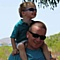Network:264If I only had one task, I wouldn't presume it would be completed at the "average" duration mark. I'd express the duration at the longest normal variance. So if the average is 6 months but 90% of all similar tasks are completed between 5-7 months, then I'd go with a 7 month duration and express a 90% confidence. (This isn't exactly how it's done, but it'll satisfy the curiosity of most stakeholders and sponsors.)

I want to avoid the "Law of Averages" fallacy, but if you had multiple tasks, then there's an even greater chance that at least one of them will extend past the average duration. If you need to calculate the real odds, then you'd need to know the typical variation of each task, on which the mean averages are based.

There'd be a very slim chance that all 10 tasks would finish no-later-than the average. That's the kind of unrealistic optimism that gets project managers into trouble.Network:911Hi Eddy, trying to read between the lines, is there enough capacity to perform all these 10 tasks in parallel? Are these 10 tasks independent so that can actually be run in parallel?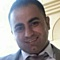Network:881Other concerns,
Will the project size/area will allow for 6 teams to work in parallel, and to store all needed materials?

Are Authority approval obtained already so you ensure no stoppage during execution.

Will all tasks will have same nature regarding the QC tests .

Detailed planning and risk analysis are critical.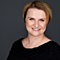Network:292Hi Eddy, I think it is ZERO. if there are no dependencies at all, it means there are 10 separate projects. If there are dependencies, so ... no chance to achieve it with so many streams. Accepting such a challenge is putting a lot of stress on PM and the team and accepting a great threat of failure. I would agree with Wade, realistic planning, good resource backup, proper contingency and open discussion with the sponsor is what is needed here.Network:1626Eddy -

Without understanding the historical variation of each parallel activity, the average for each doesn't help in determining likelihood of all completing in six months. Assuming independence of activities and probability of completing in under six months for each you could then apply basic statistics to figure out the joint probability.

KironNetwork:1174Is it 1.73% ?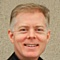Network:211To know for sure, you need to know the probability distribution for each of these paths, and then do a Monte Carlo analysis.

A simpler, but less precise approach, is to apply some basic probability. If each path is independent of the others, and each has a probability of on-time completion of 90%, then the probability that they will all complete on time is calculated by multiplying each of these probabilities. That is:

0.9 x 0.9 x 0.9 x 0.9 x 0.9 x 0.9 x 0.9 x 0.9 x 0.9 x 0.9 = 0.35 = 35%

Note that this uses 90% change of completion, not the average, as presented in the question. Using 90% is dangerous enough, let alone using the average completion dates.

Probabilities get complicated, and the paths are rarely independent, which makes matters worse. This is why you should be very wary of estimates, whether sequential or in parallel, and use some Monte Carlo analysis whenever and as much as possible in such situations.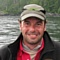Network:338I would agree with Jolanta that the probability is zero. Just because there are parallel tasks, they do not all start and finish at the same time. You have to start the framing before you start the wiring and likewise you will finish one before the other, even though you might have all 10 subcontractors working on the job for much of the time.Network:104150Six-month long activities are way too long. I suspect that further decomposing would reveal opportunities to optimize your schedule.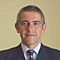Network:1899In average is not a valid statement when you talk about scheduling. On the other side the probability will depend on the point of the project life cycle you are making the estimation assuming you have made an estimation.
Page: 1 2 next>